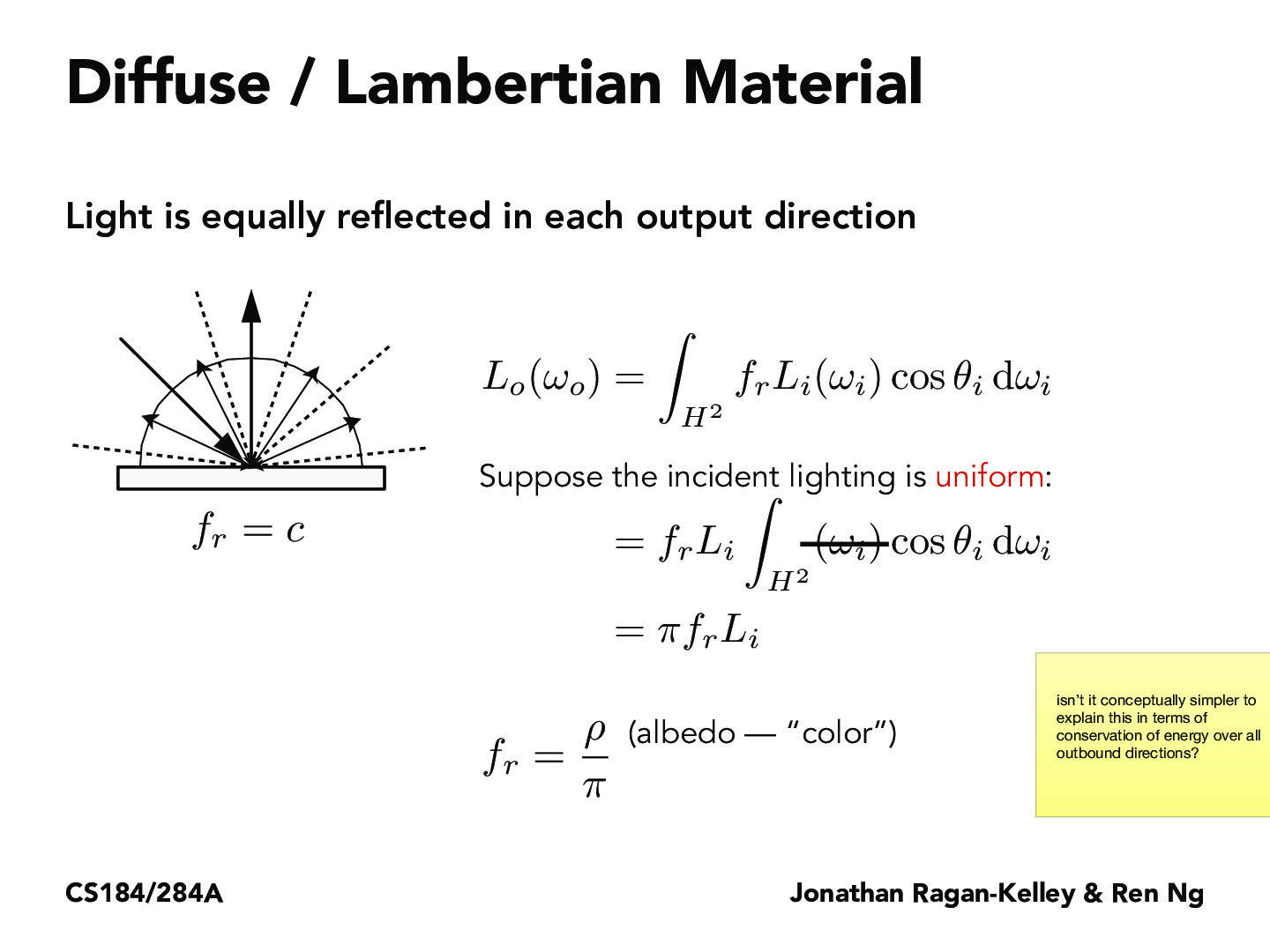You are viewing the course site for a past offering of this course. The current offering may be found here.Lecture 14: Material Modeling (10)julialuo

Here how did we get from $L_o(w_o) = \pi f_r L_i$ to $f_r = \frac{\rho}{\pi}$? I understand that the $\pi$ needs to be divided out but what exactly is albedo? Is it how much light is reflected off of the object vs absorbed?tanmayghai18

@julialuo I don't think what the slide is showing that we go from L_o(w_o) = pi * f_r * L_i to f_r = rho/pi. I think, instead, what it is trying to say is that L_o(w_o) = pi * f_r * L_i where f_r = rho/pi. Albedo refers to rho and is in reference to the surface material (lambertian). It is a measure of how reflective the surface we are looking at is and goes from 0 to 1. We divide by pi to normalize this quantity.youtuyy

As for albedo, I think it is the field reflectance in the class BSDF in our assignment 3. It is only related to the material itself, so it is a constant unchanged with the intensity of the light. I wonder what the yellow note here means? what is it explaining?kavimehta

When would we account for how light refracts differently in different mediums? Does that also affect how materials reflect light?qqqube

If f_r is not dependent on w_o or w_i, why did we include them as input parameters in the project implementation?woojinko

My guess is that f_r is dependent on w_o or w_i for different materials/mediums, though not for this diffuse/lambertian material.woojinko

This is stemming from the fact that it seemed from the bsdf file in project 3-1 that there will be a lot of different materials for which we need to write different f_r functions for in project 3-2, though I haven't checked out the specs yet.rahulmalayappan

@above, we choose fr = rho/pi because Lo/Li is the reflectance ratio, which we can also call the albedo. If we want Lo/Li = rho, then fr = rho/pi. Thus, if we specify a Lambertian surface by its albedo, we can easily find fr.

As for why we take wo and wi as inputs in the project implementation, it has to do with the abstraction shared by all BSDFs, i.e. all BSDFs take in wo and wi as input, and we shouldn't break the abstraction by "looking inside" the function and changing the interface for each material.

You must be enrolled in the course to comment